Regression Probability in Deep Networks (Bayesian Deep Learning Part II)

This post is a continuation of Explaining Dropout (Bayesian Deep Learning Part I). It is continued here for the classification problem.

Operations in the physical world are inherently uncertain, and the consequences of not understanding when to act upon the information you have are severe. Some of the most prevalent algorithms in robotics, such as particle filters for SLAM and Kalman filters for sensor fusion, are popular because they handle this uncertainty. And while no one is going to dispute the power of deep neural networks, now famous for tasks such as image classification, object detection, and text-to-speech, they do not have a well understood metric of uncertainty. You may have noticed this when Google maps happily sent you to Rome instead of Home.

The interpretation of dropout shown in the previous post casts the output of a neural network with dropout as an approximation to the output’s  true distribution. Since it is now a distribution instead of a point estimate, we can derive an expected value and variance.

The derivation for regression (taken from Yarin Gal’s thesis) is a little more straightforward, so I’ll go through that one first.

Expected Value

Since we are dealing with a probability distribution, we don’t get a single output – we must instead find the expected value of the distribution. The formula for an expected value of a continuous distribution is:Using our approximating distribution,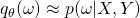, and the chain rule for probability, we get the integral:Whereis our approximating distribution,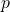is our actual distribution for input, label, and parameters (weights).andrepresent our training data. Note that since our weights are random variables, this becomes a double integral as we need to marginalize them out.

In the previous post, I mentioned that a probability prior would have to be found in order to make the objective functions match. It turns out that this prior amounts to placing independent normal distributions across each weight, with a specific standard deviation (). We can then reformulate:The expected value of a Gaussian distribution is just its mean, and the mean is determined by our network output (the parameter),. So if we integrate out our, we find the expected value with one more parameter to marginalize out.If we evaluate every possible combination of weights and average them (since the more likely output will occur more frequently), we can estimate this integral. This is called Monte Carlo Integration.

Since the weights are Bernoulli distributed, a combination of weights is simply one potential set of activations. Or, pragmatically, a forward pass with dropout enabled. Each of these forward passes results is a single Monte Carlo sample, which leaves us with the final estimator:

(1)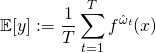For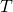samples. As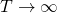, we approach the true expected value.

Variance

While performing inference time dropout improves network performance slightly, the  gain is not sufficient to justify performing multiple inferences for Monte Carlo integration. The real reason this process is valuable is its ability to estimate variance.

(2)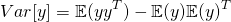I note that my version of the equation differs slightly from the one in Gal’s thesis. The only reason for this is that I more traditionally see covariance written asfor column vectors, while Gal is performs his derivation with row vectors.is known from previous, but we still need to figure out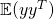. Conveniently, the procedure is much the same as for expected value. We start with the expected value formula, usinginstead of. We note thatandhave the same probability density function.We note that the integral with respect tois just the expected covariance, so we can rearrange 2 such that it represents expected variance.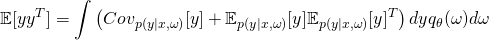Since we know, and the covariance, this becomes:The term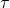is actually a characteristic of the network itself. It’s tied to the weight-decay parameter and dropout rate. I haven’t needed to dig my teeth into this yet, so I can’t provide a good explanation of that parameter. HOWEVER, the definition of this term is such that if your network has no weight decay parameter, it can be ignored (as,).

Since ourterm is not dependent on, andintegrates to 1, we can pull it out of the integral.  Monte Carlo integration is performed on the remaining term in the same manner as 1. This results in the final unbiased estimator for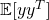: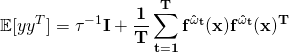And substituting this estimate back into 2 we end up with our final estimator for variance:This site uses Akismet to reduce spam. Learn how your comment data is processed.